Skip to main content

# 5.3: HMBC and HMQC Spectra

Just as COSY spectra show which protons are coupled to each other, HMBC (and the related HMQC) give information about the relative relationships between protons and carbons in a structure.

In an HMQC spectrum, a 13C spectrum is displayed on one axis and a 1H spectrum is displayed on the other axis. Cross-peaks show which proton is attached to which carbon. COSY spectra show 3-bond coupling (from H-C-C-H), whereas HMQC shows a 1-bond coupling (just C-H).

Take a look at n-hexylbenzene. There are lots of similar positions in this structure, so it may be hard to tell peaks apart in either the proton or carbon NMR spectrum.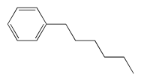Indeed, the 1H spectrum has some unique peaks but also a couple of large multiplets.Similarly, there are a couple of carbons that show up at the same place in the 13C NMR spectrum.An HMQC experiment will help spread things out into two dimensions. Looking along the x axis, we can see that several peaks were clustered together near 7 ppm and near 1.3 ppm. Even looking along the y axis, we can see a couple of carbon peaks have separated out from each other around 33 ppm.An HMBC spectrum looks very similar to an HMQC spectrum, except that it shows 2-bond, 3-bond or sometimes even 4-bond coupling (not H-C, but H-C-C or H-C-C-C or even H-C-C-C-C). Instead of seeing which carbon is directly attached to a hydrogen, we see which carbon is next to that one, so that we begin to see how the molecule connects.

Exercise $$\PageIndex{1}$$

Analyse the data to determine which of the two isomers (below) we are dealing with.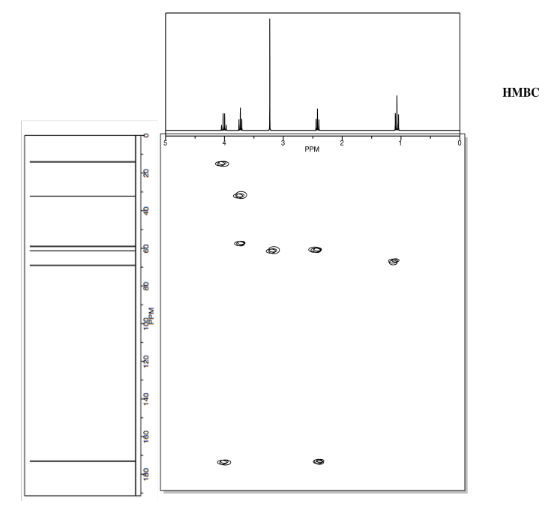AnswerExercise $$\PageIndex{2}$$

Analyze the data to determine which of the two isomers (below) we are dealing with.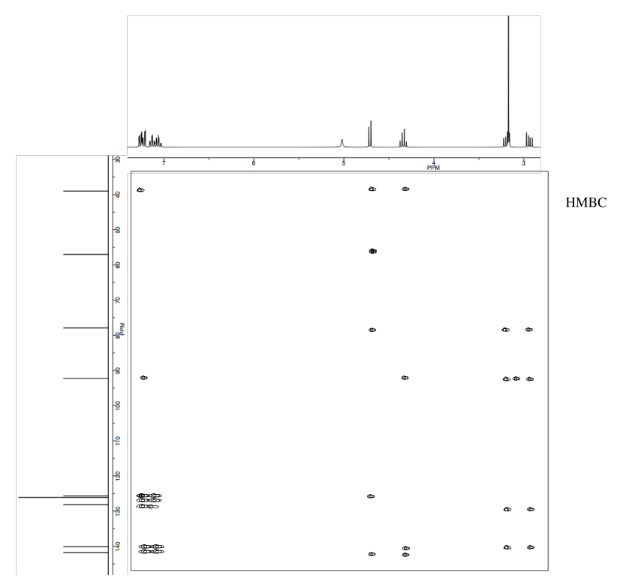AnswerExercise $$\PageIndex{3}$$

Analyse the data to determine the structure of the compound.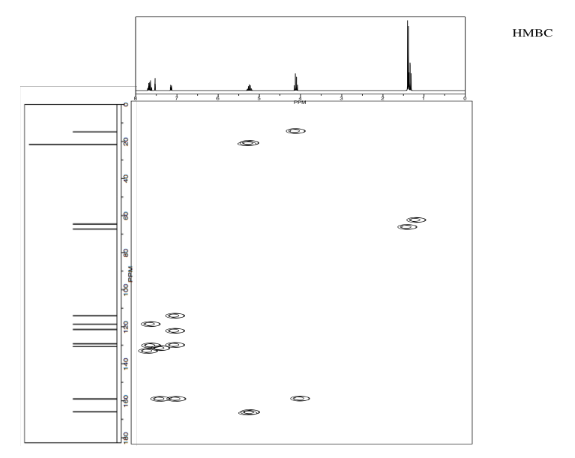AnswerExercise $$\PageIndex{4}$$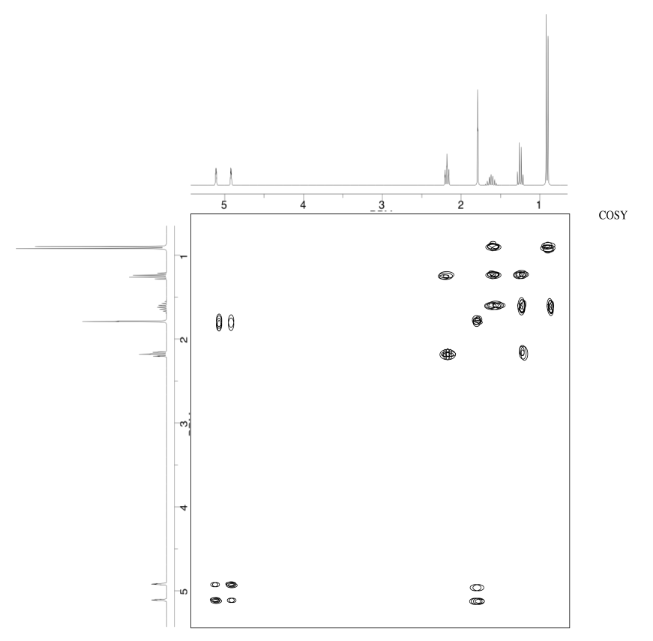AnswerExercise $$\PageIndex{5}$$AnswerExercise $$\PageIndex{6}$$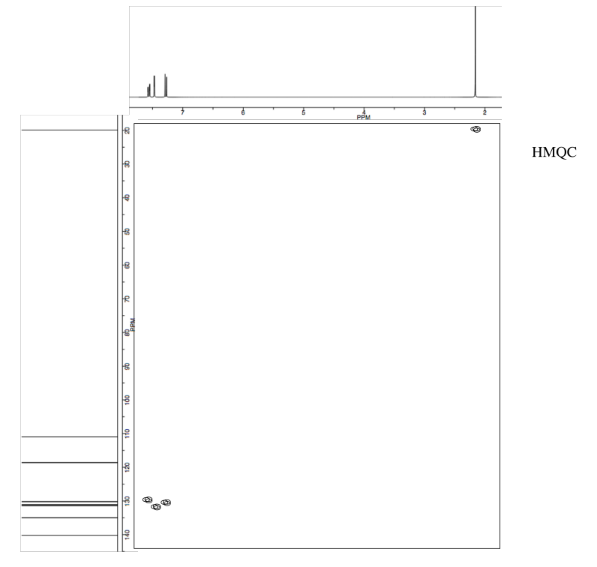AnswerExercise $$\PageIndex{7}$$AnswerExercise $$\PageIndex{8}$$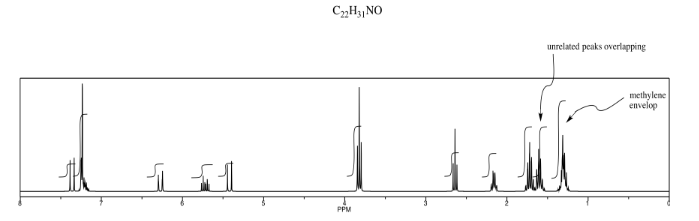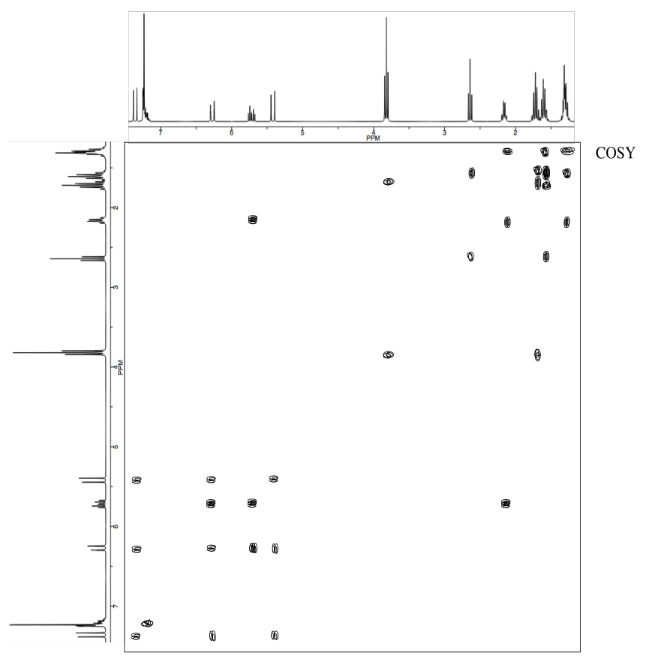Answer*Sources:

Selected IR spectra from SDBS (National Institute of Advanced Industrial Science and Technology, Japan, Spectral Database for Organic Compounds, http://sdbs.db.aist.go.jp/sdbs/cgi-bin/cre_index.cgi, accessed December, 2015).

1H NMR, 13C NMR, HMBC and HMQC spectra simulated.